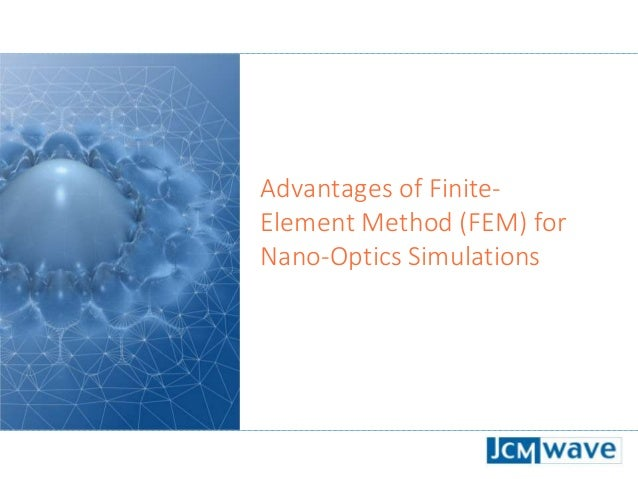Successfully reported this slideshow.Upcoming SlideShare
×

# Advantages of Finite-Element Method for Nano-Optics Simulations

Brief explanation of some advantages of the Finite-Element Method (FEM) for simulations in photonics and nano-optics. Benchmarks against FDTD, RCWA and other FEM solvers show the exceptionally short computation times of the higher-order FEM method implemented in JCMsuite.

• Full Name
Comment goes here.

Are you sure you want to Yes No### Advantages of Finite-Element Method for Nano-Optics Simulations

1. 1. Advantages of Finite- Element Method (FEM) for Nano-Optics Simulations
2. 2. 2 Typical simulations in nano-optics Compute solution to Maxwell‘s equations in frequency-domain: 𝛻 × 𝜇(𝐫)−1 𝛻 × 𝐄 𝐫, 𝜔 − 𝜔2 𝜖 𝐫 𝐄 𝐫, 𝜔 = iω𝐉imp 𝐫, 𝜔 𝜇 𝐫 , 𝜖(𝐫): Spatially dependent permeability and permittivity of the setup. 𝐉imp (𝐫): Current impressed by an external source (plane-wave illumination, laser beam, dipole emitter, fiber mode, etc.) 𝜔: Harmonic frequency of the source term. 𝐄 𝐫, 𝜔 : Time-harmonic electric field strength. The time-dependent field is given as 𝐄 𝐫, 𝑡 = ℜ{𝐄 𝐫, 𝜔 ⋅ 𝑒−𝑖𝜔𝑡}.
3. 3. 3 Example: Add-drop multiplexer (integrated optics) In-coupling waveguide mode, i.e. 𝐉imp(𝐫, 𝜔) Drop port Add port Through port Wave guide: 𝜖1 = 12.1𝜖0 Substrate: 𝜖2 = 2.3𝜖0 Electric field intensity 𝐄 𝐫, 𝜔 2 fulfilling 𝛻 × 𝜇(𝐫)−1 𝛻 × 𝐄 𝐫, 𝜔 − 𝜔2 𝜖 𝐫 𝐄 𝐫, 𝜔 = iω𝐉imp 𝐫, 𝜔
4. 4. 4 How to solve Maxwell’s equations?  RCWA (rigorous coupled wave analysis): The geometry is discretized into individual layers. The diffraction of incident plane waves at the structure is calculated. [Wikipedia]  FDTD (finite difference time-domain method): The geometry is discretized into uniform patches (squares, cubes). The equations are solved in a time and space discrete manner. [Wikipedia]  FEM (finite element method): The geometry is discretized into variable shapes like triangles, tetrahedrons, prisms (solution: next slide). Various methods are used to solve Maxwell’s equations rigorously, e.g.: FDTD
5. 5. 5 1. Choose computational domain with appropriate boundary condition and sub-divide the geometry into patches 2. Expand the electric/magnetic fields with local ansatz functions which are defined on the triangles/tetrahedrons/prisms etc. and plug into weak formulation of Maxwell‘s equations 3. Solve sparse matrix equation with fast numerics FEM Recipe
6. 6. 6 The flexibility of FEM Due to its flexible geometry discretization, FEM can be applied to various geometries and complex shapes.
7. 7. 7 Examples of shape discretization Cavity for sensing applications Helix nanoantenna Circular grating resonator for optical switching Smooth vs. rough surface
8. 8. 8 The efficiency of FEM The precision of the FEM solution can be locally adapted. This leads to highly accurate results at short computation times.
9. 9. 9 Hp-Finite Element Method FEM numerical parameters: h - triangle size, p - polynomial order Suitable non-uniform combination of p and h refinements leads to superior convergence [Babuska, 1992]
10. 10. 10 Comparison of convergence speed FEM RCWA FDTD Comparison: FEM vs. FDTD vs. RCWA Benchmark Problem: Rigorous Mask Simulation for Lithography FEM faster and more accurate by orders of magnitude [Benchmark of FEM, Waveguide and FDTD Algorithms for Rigorous Mask Simulation. Proc. SPIE 5992, 368, 2005.]
11. 11. 11 Comparison of convergence speed (2) Comparison: The FEM solver of JCMsuite is compared to other commercial and non-commercial FEM-solvers (see reference for a detailed description) Benchmark problem: Complex eigenfrequencies of the modes of a 2D plasmonic crystal composed of a periodic array of metallic squares in air. [Quasinormal mode solvers for resonators with dispersive materials. JOSA A 36, 686, 2019.]
12. 12. 12 Resources  Description of FEM software JCMsuite  Free trial download of JCMsuite  Getting started with JCMsuite  Benchmark of rigorous methods for electromagnetic field simulation  Benchmark of quasinormal mode solvers